# Dividing monomials

 Dividing monomials is reducing fractions with variables. The easiest way to do this is to write out (expand) both the numerator and the denominator. Then, cross out each pair of numbers or variables that appears both in the numerator and the denominator. Here's a few examples: 1)2)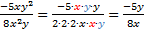3)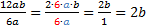4)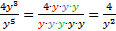Practice: 1)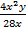2)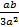3)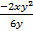4)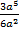5)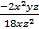Answers: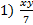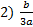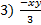4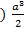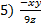Related Links: Math Algebra Polynomials Algebra Topics